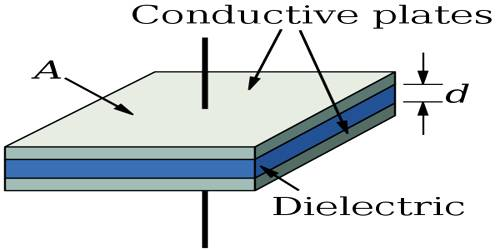# Dielectrics

Dielectrics

Any non-conducting or insulating medium between any two point charges keeps, the charges isolated. This type of medium is called dielectric medium.

Examples of dielectric materials are glass, ebonite, rubber, oil, wax etc. The principal difference between conductor and dielectrics is that there are no free electrons in dielectrics. If some extra charges are given to a dielectric material, they remain accumulated in the region where these are given. Question is what will be the value of the resultant electric field if the dielectric material is placed in an external electric field. Normally, the resultant field at any point inside Dielectrics is less than the external field, but the field in the dielectric never becomes zero like that in a conductor.

Force acting between two point charges in vacuum or in air medium has been obtained as,

F0 = (1 / 4πε0) (q1q2/r2) … … … (1)

In any other insulating medium this force is,

F = (1 / 4πε) (q1q2/r2) … … … (2)

Dividing equations (1) by (2), we get,

F0/F = ε/ε0 = ε

so, εr = ε/ε0

= F0/F = [Force in air or vacuum / Force at insulating or dielectric medium] at same distance.

This ε, is called the dielectric constant id the medium. Sometimes, it is also called relative permittivity. From the above discussion, we can give the following definition of dielectric constant.Definition: The ratio of the forces between two charges at a particular distance in the dielectric medium and that in air or vacuum is called the dielectric constant.

Since εr is a ratio, so it has no unit.

Dielectrics are insulators, plain and simple. The two words refer to the same class of materials, but are of different origin and are used preferentially in different contexts.